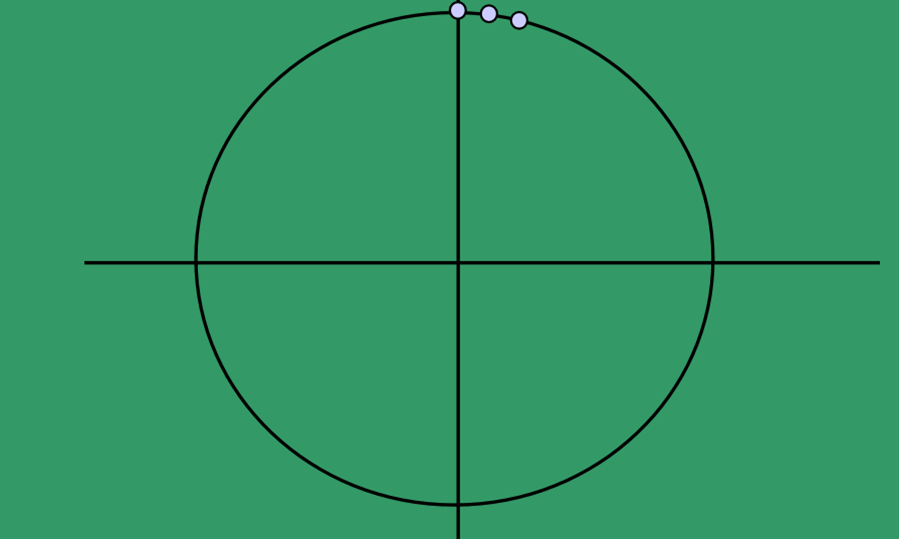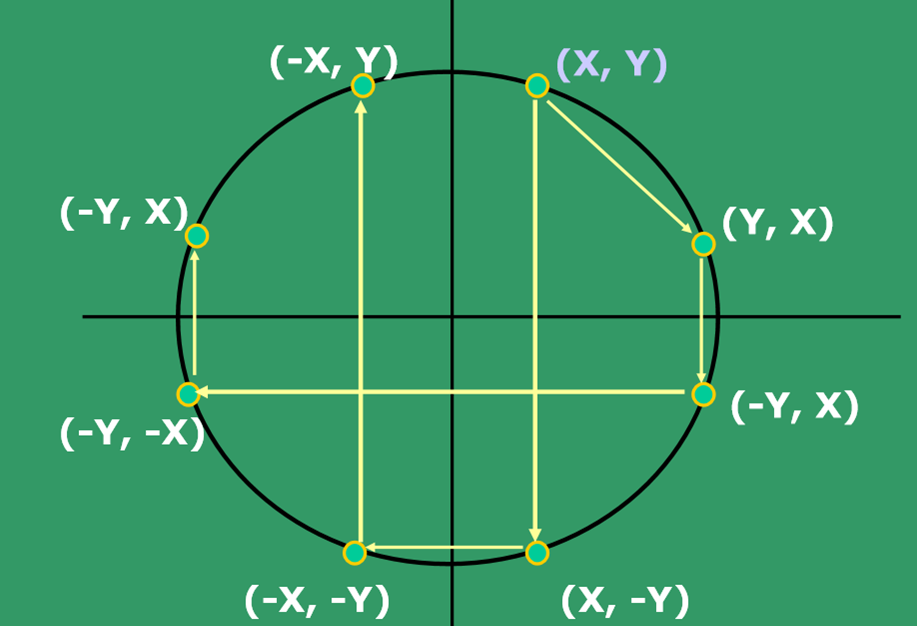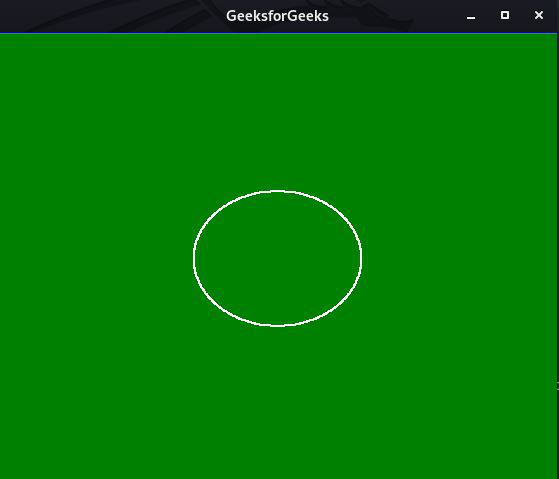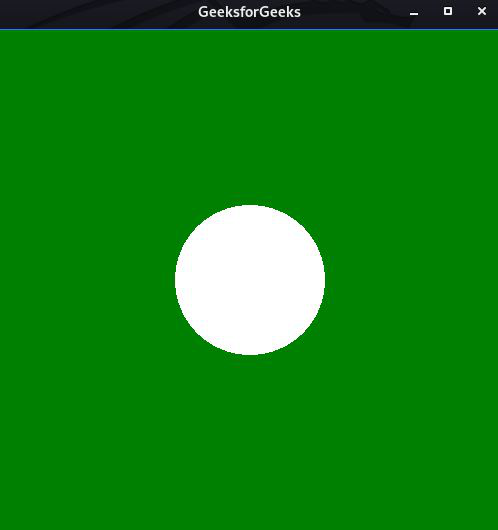Open in App
Not now

# Draw circle using polar equation and Bresenham’s equation

• Last Updated : 18 Dec, 2020

In this article, we will discuss how to draw circles using Bresenham Equation and Polar Equation.

### Circle Drawing Algorithm

It is not easy to display a continuous smooth arc on the computer screen as our computer screen is made of pixels organized in matrix form. So, to draw a circle on a computer screen it should always choose the nearest pixels from a printed pixel so as they could form an arc.• Consider circles centered at the origin with integer radii.
• Can apply translations to get non-origin centered circles.
• Equation of circle is given by:

x2 + y2 =  R2
y = +/-sqrt(R2-x2)

• The given equation can be written as:

F(x, y)= x2+ y2-R2=0
5.

• Use of Symmetry: Only need to calculate one octant. One can get points in the other 7 octants as follows:
• Plotpoint(x, y)
• Plotpoint(y, x)
• Plotpoint(x, -y)
• Plotpoint(-y, x)
• Plotpoint(-x, -y)
• Plotpoint(-y, -x)
• Plotpoint(-x, y)
• Plotpoint(-y, x)

### Circle Drawing Using Bresenham Equation

Bresenham Equation uses the key feature of a circle that is highly symmetric. So, for the whole 360 degrees circle, divide it into 8-parts each octant of 45 degrees. In order to that, the idea is to use Bresenham’s Circle Algorithm for the calculation of the locations of the pixels in the first octant of 45 degrees. It assumes that the circle is centered on the origin. So for every pixel (x, y) draw a pixel in each of the 8 octants of the circle as shown below:In Bresenham’s Algorithm at any point (x, y) we have two options either to choose the next pixel in the east i.e., (x + 1, y) or in the south-east i.e., (x + 1, y – 1). And this can be decided by using the decision parameter d as:

• If d > 0, then (x + 1, y – 1) is to be chosen as the next pixel as it will be closer to the arc.
• Else (x + 1, y) is to be chosen as the next pixel.

Below is the algorithm for Bresenham Equation:

• F(x, y) = x2 + y2 = 0 Point lies on the circle.
• F(x, y) > 0 Point lies outside the circle.
• F(x, y) < 0 Point lies inside the circle.
• If d >= 0 then update x as (x + 1) and y = (y – 1) which gives new d
• If d < 0 then update x as (x + 1) which gives the new value of d

## C

 `// C program for the above approach ` `#include ` `#include ` `#include ` `#include ` ` `  `int` `xo, yo, r; ` ` `  `// Function to display the circle using ` `// the above algorithm ` `void` `Display(``void``) ` `{ ` `    ``glClear(GL_COLOR_BUFFER_BIT); ` ` `  `    ``// Color of printing object ` `    ``glColor3f(1, 0, 0); ` ` `  `    ``// Giving the size of the point ` `    ``glPointSize(2); ` ` `  `    ``int` `x = 0; ` `    ``int` `y = r; ` `    ``float` `p = 5 / 4 - r; ` ` `  `    ``glColor3f(1, 0, 0); ` ` `  `    ``// Starting of drawing the circle ` `    ``glBegin(GL_POINTS); ` ` `  `    ``while` `(y > x) { ` `        ``if` `(p < 0) { ` ` `  `            ``// Increment x to x+1 ` `            ``x++; ` `            ``p = p + 2 * x + 1; ` `        ``} ` `        ``else` `{ ` ` `  `            ``// Increment x to x+1 ` `            ``// and decrease y to y-1 ` `            ``y--; ` `            ``x++; ` `            ``p = p + 2 * (x - y) + 1; ` `        ``} ` ` `  `        ``// Draw the coordinates ` `        ``glVertex2d(x + xo, y + yo); ` `        ``glVertex2d(-x + xo, y + yo); ` `        ``glVertex2d(x + xo, -y + yo); ` `        ``glVertex2d(-x + xo, -y + yo); ` `        ``glVertex2d(y + yo, x + xo); ` `        ``glVertex2d(-y + yo, x + xo); ` `        ``glVertex2d(y + yo, -x + xo); ` `        ``glVertex2d(-y + yo, -x + xo); ` `    ``} ` ` `  `    ``glEnd(); ` ` `  `    ``// Its empties all the buffer ` `    ``// causing the issue ` `    ``glFlush(); ` `} ` ` `  `// Driver Code ` `int` `main(``int` `argc, ``char``** argv) ` `{ ` `    ``printf``(``"X-coordinate Y-coordinate radius:"``); ` `    ``scanf``(``"%d %d %d"``, &xo, &yo, &r); ` `    ``glutInit(&argc, argv); ` `    ``glutInitDisplayMode(GLUT_SINGLE | GLUT_RGB); ` ` `  `    ``// Assigning the size of window ` `    ``glutInitWindowSize(1000, 1000); ` ` `  `    ``// Assign the position of window ` `    ``// to be appeared ` `    ``glutInitWindowPosition(100, 100); ` ` `  `    ``// Defining the heading of the window ` `    ``glutCreateWindow(``"GeeksforGeeks"``); ` ` `  `    ``// Backgronnd Color ` `    ``glClearColor(1, 1, 1, 1); ` ` `  `    ``// limit of the coordinate points ` `    ``gluOrtho2D(-500, 500, -500, 500); ` ` `  `    ``// Calling the function ` `    ``glutDisplayFunc(Display); ` ` `  `    ``glutMainLoop(); ` ` `  `    ``return` `0; ` `}`

Output:Time Complexity: O(N)
Auxiliary Space: O(1)

### Circle Using Polar Equation

In the Polar Equation system, the idea is to think of a clock with one hand. Move out a distance r, sometimes called the modulus, along with the hand from the origin, then rotate the hand upward (counterclockwise) by an angle θ to reach the point. Below is the algorithm for the Polar Equation:

1. Initialize the variables rad, center(x0, y0), index value or increment value i, and define a circle using polar coordinates θ_end = 100.
2. If θ_end < θ, then exit from the loop.
3. Find the value of x as rad*cos(angle) and y as rad*sin(angle).
4. Plot the eight points, found by symmetry i.e., the center (x0, y0) at the current (x, y) coordinates.
•  Plot (x + xo, y + yo)
• Plot (-x + xo, -y + yo)
• Plot (y + xo, x + yo)
• Plot (-y + xo, -x + yo)
• Plot (-y + xo, x + yo)
• Plot (y + xo, -x + yo)
• Plot (-x + xo, y + yo)
• Plot (x + xo, -y + yo)
5. Increment the angle by i*2*(M_PI/100).

Below is the program to implement the above approach:

## C

 `// C program to demonstrate circle ` `// drawing using polar equation ` `#include ` `#include ` `#include ` `#include ` `float` `xo, yo, rad; ` ` `  `// Function to display the circle ` `void` `display() ` `{ ` `    ``glClear(GL_COLOR_BUFFER_BIT); ` ` `  `    ``// Color of printing object ` `    ``glColor3f(1, 1, 1); ` ` `  `    ``float` `angle; ` ` `  `    ``// Start to drawing the circle ` `    ``glBegin(GL_POLYGON); ` ` `  `    ``for` `(``int` `i = 0; i < 100; i++) { ` ` `  `        ``// Change the angle ` `        ``angle = i * 2 * (M_PI / 100); ` `        ``glVertex2f(xo + (``cos``(angle) * rad), ` `                   ``yo + (``sin``(angle) * rad)); ` `    ``} ` ` `  `    ``glEnd(); ` ` `  `    ``// Its empties all the buffer ` `    ``// causing the issue ` `    ``glFlush(); ` `} ` ` `  `// Driver Code ` `int` `main(``int` `argc, ``char``** argv) ` `{ ` `    ``glutInit(&argc, argv); ` `    ``printf``(``"Enter x y radius "``); ` `    ``scanf``(``"%f %f %f"``, &xo, &yo, &rad); ` ` `  `    ``glutInitDisplayMode(GLUT_SINGLE | GLUT_RGB); ` ` `  `    ``// Assigning the size of window ` `    ``glutInitWindowSize(500, 500); ` ` `  `    ``// Assign the position of window ` `    ``// to be appeared ` `    ``glutInitWindowPosition(200, 200); ` ` `  `    ``// Defining the heading of the window ` `    ``glutCreateWindow(``"GeeksforGeeks"``); ` ` `  `    ``// Backgronnd Color ` `    ``glClearColor(0, 1, 0, 1); ` ` `  `    ``// limit of the coordinate points ` `    ``gluOrtho2D(-500, 500, -500, 500); ` ` `  `    ``// Calling the function ` `    ``glutDisplayFunc(Display); ` ` `  `    ``glutMainLoop(); ` ` `  `    ``return` `0; ` `}`

Output:Time Complexity: O(N)
Auxiliary Space: O(1)

My Personal Notes arrow_drop_up
Related Articles